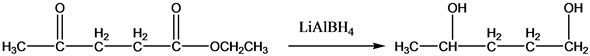Problem

# Show how you would accomplish the following transformations. You may use any additional...

Show how you would accomplish the following transformations. You may use any additional reagents you need.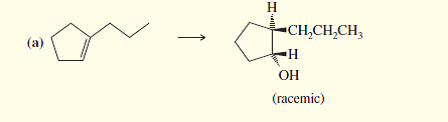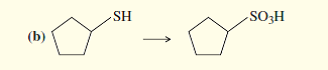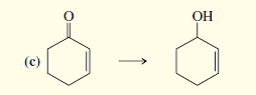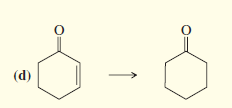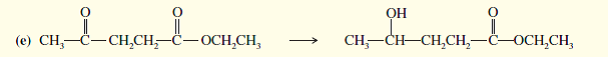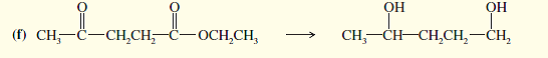#### Step-by-Step Solution

Solution 1

The transformations are given below--

(a).b)

The transformations are given belowThe transformations are given below--

(c).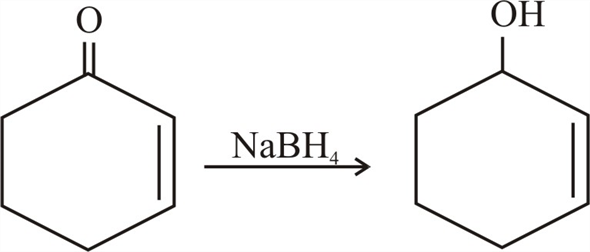The transformations are given below--

(d).The transformations are given below--

(e).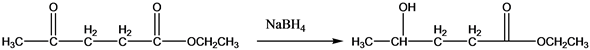The transformations are given below--

(f).